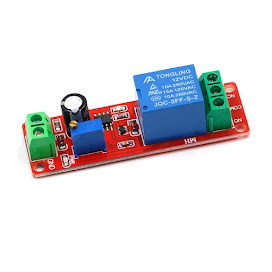## Saturday, September 25, 2021

### MATLAB - Family of Curves (Vectorization)

Linear Signals and System, matrix operation fundamentals. This example illustrates a techniques called vectorization. Algorithm vectorization uses matrix and vector operations to avoid manual repetition and loop structures. With simultaneous creation of curves one can maximize the use of matrix applications.
The result here is a 201 x 11 matrix with identical columns.

%% Matrix Operations - Family of Curves
r = [1 0 0]
A = [2 3;4 5;0 6]
c = r' B = [c A]
B(1,2)
B(1:2,2:3)
B(2,:)
A = [1 -2 3;-sqrt(3) 1 -sqrt(5);3 -sqrt(7) 1];
y = [1;pi;exp(1)];
x = inv(A)*y x1 = det([y,A(:,2:3)])/det(A) alpha = (0:10);
t = (0:0.001:0.2)';
T = t*ones(1,11);
H = exp(-T*diag(alpha)).*sin(2*pi*10*T+pi/6);
plot(t,H); xlabel('t'); ylabel('h(t)');
[R,P,K] = residue(B,A)
[R,P,K] = residue([1 0 0 0 0 pi],[1 -sqrt(8) 0 sqrt(32) -4]);
R.', P.', K title('Family of Curves - Vectorization')

Try this code out to graph this figure! Modify freely and comment on this post if it helps. Thanks for reading.